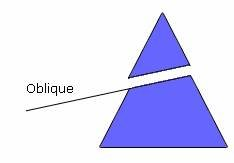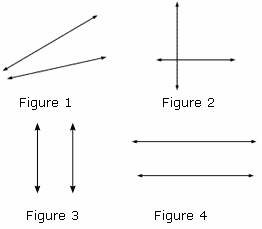Related Links

## Definition Of Oblique

The lines that are drawn at an angle (not 900) to the horizon are called Oblique Lines.

### More About Oblique

Oblique lines are neither vertical nor horizontal.

### Example of ObliqueThe above figure shows a triangle being cut by an oblique line.

### Solved Example on Oblique Prism

#### Ques: Identify the oblique lines from the figures shown.##### Choices:

A. Figure 1
B. Figure 2
C. Figure 3
D. Figure 4
Correct Answer: A

#### Solution:

Step 1: Here, the lines in figure 1 are neither vertical nor horizontal.
Step 2: So, the lines in figure 1 are oblique lines.